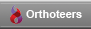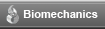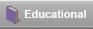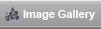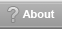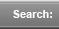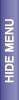# Material Behavior

Newton by William Blake (1757-1827)

The principles of Biomechanics rest on the three laws of motion set by Sir Issac Newton.

1st Law : An object at rest will remain at rest or an object in motion will remain in a straight line with constant velocity if the net force acting in the object is zero.

2nd Law : An object on which force is exerted will move in the direction of net force and the acceleration will be proportional to the net force

3rd Law : Every action has an opposite and equal reaction

The quantities described in biomechanics are either Scalar or Vector.

A scalar quantity is one that is defined only by its magnitude. Examples include: mass, work. A vector, on the other hand, has magnitude as well as direction, examples include force,velocity etc. Vector is represented by an arrow. Its length represents the magnitude, orientation the line of action and arrowhead the direction and sense of vector.

Force : Force is action of one body on another. It is a vector. The line of action of a muscle is defined as passing through the centre of muscle. Since it is a vector, a force has a magnitude, a direction and a point of application.

Force= massÃ—acceleration (from Newton's 2nd law)

kg.m/sec 2 =kgÃ—m/sec 2 This unit of force is Newton (N)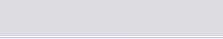Orthoteers is a non-profit educational resource. Click here for more details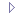Loading of StructuresMaterial BehaviorMaterial Properties